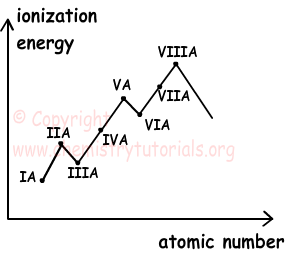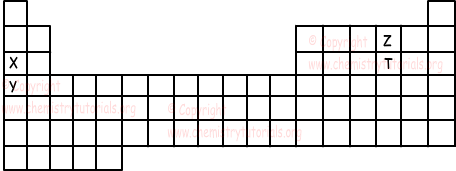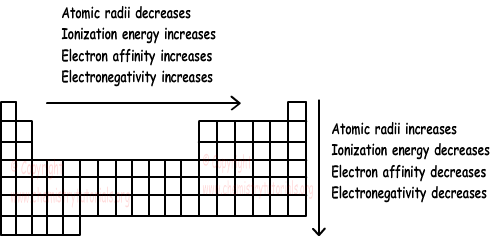# Periodic Properties of Elements with Examples

Periodic Properties of Elements with Examples

Atomic radius of elements decreases as we go from left to right in periodic table. Reason is that; atomic number of elements increase from left to right in same period, thus increase in the number of protons causes increases in attraction of electrons by protons. On the contrary, in same group, as we go from top to bottom, atomic radius of elements increase. Since number of shells increase in same group from top to bottom, attraction of electrons by protons decrease and atomic radius increase.

Example: Find relation between atomic radius of elements 3X, 11Y and 5Z.

We first find the locations of elements in periodic table.

3X:1s22s1 2. period I A group

11Y:1s22s22p63s1 3. period and I A group

5Z:1s22s22p1 2. period and III A group.

I A      III A

1. period         X         Z

2. period         Y

Since atomic radius increase from right to left and top to bottom;

Y>X>Z

2) Ionization Energy:

Energy required to remove an electron from atoms or ions is called ionization energy . Energy required to remove first valence electron is called first ionization energy, energy required to remove second valence electron is called second ionization energy etc. Following reactions show this process;

X + IE1→X+ + e-

X+ + IE2→X+2 + e-

X+2 + IE3→X+3 + e-

Increasing in the attraction force applied by nucleus to electrons makes difficult to remove electrons from shells. Second ionization energy is larger than first ionization energy, second ionization energy is larger than third ionization energy. We can say that;

IE1<IE2<IE3<….

When electrons are removed from atom, attraction force per electron increases, thus removing electron from atom becomes more difficult. Atoms having electron configuration ns2np6 has spherical symmetry property and removing electron is difficult and ionization energy is high. Moreover, atoms having ns2np6ns1 has lower ionization energy, because removing one electron from these atoms make them noble gas and more stable. Thus, it is easy to remove electron from them. For example;

10Ne: 1s22s22p6 and

11Na: 1s22s22p63s1

IENe>IENa

Knowing sequential ionization energies of atom, helps us to find number of valence electrons of atoms. Examine following example;

IE1 IE2 IE3 IE4 IE5

176    347     1850     2520     3260

Increase in second to third ionization energy is greater than others, thus atom has 2 valence electrons.

Example:

Na(gas) + IE1→Na++ e-

Na(gas) + IE2→Na+2 + 2e-

Na(solid) + IE3→Na+ + e-

Na+(solid) + IE4→Na+2+ e-

Which one of the following statements related to chemical equations given above are false.

I. E1 is the first ionization energy of Na

II. E3>E1

III. E2 is second ionization energy of Na

IV. E4>E1

V. E2=E1+E4

First ionization energy is the energy required for removing one electron from neutral atom in gas state. I is true.

E3 is the sum of energies E1 and sublimation energy. Thus, E3>E1 II is true

Second ionization energy is the energy required for removing one electron from +1 charged ion in gas state.Thus, III is false.

E4 is the second ionization energy and E1 is first ionization energy. Thus; E4>E1 IV is true

Na(gas) + IE1→Na++ e-

Na+(solid) + IE4→Na+2 + e-

——————————————

Na(gas) + (E1+E4)→Na+2(gas)+2e-

So; E2=E1+E4 V is true

Changes of Ionization Energy in Periodic Table;

I A<III A<II A<IV A<VI A<V A<VII A<VIII A

Since II A and  V A has spherical symmetry property they have greater ionization energies then III A and VI A. Graph given below shows relation between ionization energy and atomic number.3) Electron Affinity:

If an electron is added to neutral atom in gas state, energy is given off. We call this energy “electron affinity “.Following chemical equation shows this process.

X(gas) + e- → X-(gas) + E

In general, electron affinity increases as we go from left to right in period. On the contrary, electron affinity decreases in a group from top to bottom.

4) Electronegativity:

In a chemical bond, electron attraction capability of atoms is called electronegativity. From left to right in period electronegativity increases and from top to bottom in a group electronegativity decreases. Since noble gases do not form chemical bonds, we can not talk about their electronegativity.

5) Metal-Nonmetal Property:

Capability of giving electron is called metal property and capability of getting electron is called non metal property of elements. Moving in period from left to right, metal property increases and  non metal property decreases. In a group of metals, from top to bottom metal property increases. In a groups of non metals, from top to bottom non metal property of atoms decreases.

Example: Which one of the following statement is true related to given elements in the periodic table below.I. Metal property of X is larger than Y, Z and T.

II. Atomic radius of Z is larger than X, Y and T.

III. Ionization energy of T is larger than IE of X.

IV. The most electronegative element is Y.

Metal property increases from right to left and top to bottom. Thus Y is the most metallic element. I is false.

Atomic radii increases from right to left and top to bottom. Thus Y has greater atomic radii. II is false.

Ionization energy increases from left to right in same period. Thus, IET>IEX. III is true.

Electronegativity increases from left to right and bottom to top. Z is the most electronegative element.

Summary of periodic properties is given in the picture below.Periodic Table Exams and Problem Solutions